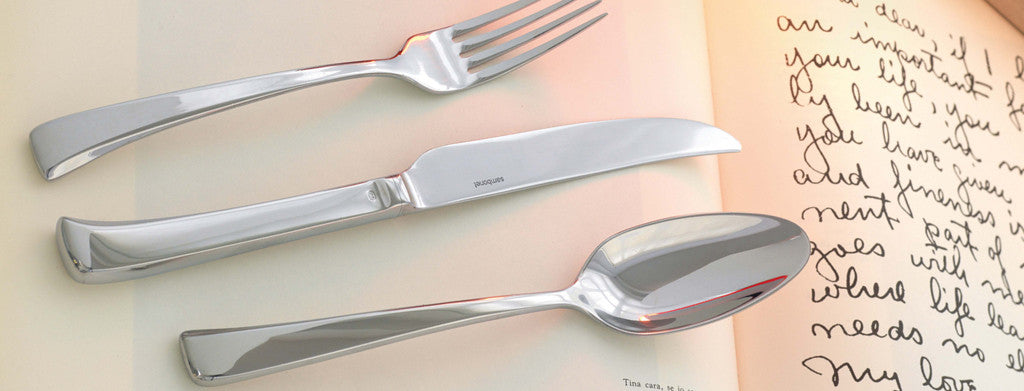Shopping Cart

### Refine

View all

#### Price# Cutlery£1.79 each (ex VAT)£1.79 each (ex VAT)£1.73 each (ex VAT)£0.27 each (ex VAT)£0.48 each (ex VAT)£6.68 each (ex VAT)£6.68 each (ex VAT)£21.34 each (ex VAT)£21.34 each (ex VAT)£6.80 each (ex VAT)£11.21 each (ex VAT)£21.34 each (ex VAT)£0.30 each (ex VAT)£0.27 each (ex VAT)£0.91 each (ex VAT)£0.48 each (ex VAT)£0.47 each (ex VAT)£0.85 each (ex VAT)£0.47 each (ex VAT)£0.67 each (ex VAT)£0.51 each (ex VAT)£6.68 each (ex VAT)£1.79 each (ex VAT)£2.02 each (ex VAT)£1.73 each (ex VAT)£22.40 each (ex VAT)£20.97 each (ex VAT)£20.65 each (ex VAT)£20.65 each (ex VAT)£21.22 each (ex VAT)£20.12 each (ex VAT)£12.30 each (ex VAT)£12.30 each (ex VAT)£20.22 each (ex VAT)£6.80 each (ex VAT)£1.97 each (ex VAT)£4.54 each (ex VAT)£0.48 each (ex VAT)£0.91 each (ex VAT)£0.48 each (ex VAT)£0.91 each (ex VAT)£0.47 each (ex VAT)£0.46 each (ex VAT)£0.85 each (ex VAT)£0.46 each (ex VAT)£0.30 each (ex VAT)£0.30 each (ex VAT)£0.48 each (ex VAT)